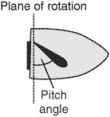# pitch angle

Also found in: Dictionary, Wikipedia.

## pitch anglei. The angle between the chord line of a propeller blade and the plane of rotation.
ii. The angle between the rotor plane and the chord line.
iii. The angle between the chord line and a line that is horizontal or parallel to the surface of the earth.
References in periodicals archive ?
net height (m) Ground gear Port roll angle (deg.) Door x Scope Port pitch angle (deg.) Door x Ground gear Starboard roll angle (deg.) Scope x Ground gear Starboard pitch angle (deg.) Door x Ground gear x Scope Trawl catch rates Ranked weight CPUE (kg/[km.sup.2]) Ranked number CPUE (no./[km.sup.2])
In our application longitudinal autopilot is tracking 5 degrees of pitch angle and lateral autopilot is tracking 5 degrees of roll angle.
8 shows the curve of battery pack pitch angle, X coordinate axle represents time (s).
(iv) Pitch-down descend phase: the phase from leaving the lowest step until the pitch angle of the robot body, [[theta].sub.p], evenly matches the lower floor
It is inferred from Figure 9, due to the reliable refusion information as the measurements ([[phi].sub.fusion], [[theta].sub.fusion]), selected benchmark algorithms based on QUEST and the proposed method have similar low dynamic performance for estimating the roll and pitch angle. The RMS of roll and pitch in IKF is 0.31[degrees] and 0.33[degrees], respectively.
The specified region n is also a system state in an expected pitch angle or radial impact velocity range of the subsatellite.
Taken with a roll tilt angle of 0 degree and roll pitch angle of 79.63 degrees, the final image was post-processed to highlight the crescent aspect of the moon.
To define the participants' position or activity, three variables from the accelerometers were derived: Euclidean Norm Minus One (ENMO), thigh pitch (elevation) angle: and lower leg pitch angle. ENMO was calculated using the following formula: ENMO = ([square root of ([x.sup.2] + [y.sup.2] + [z.sup.2]))] - 1, where x, y and z refer to the acceleration in g detected along the x, y, and z axes, respectively.
The missile uses vertical launching mode, The angular displacement of the missile around the inertial coordinate system x-, y-, and z-axis is defined as the yaw angle [[theta].sub.x], pitch angle [[theta].sub.y], and roll angle [[theta].sub.z].
where [[rho].sub.w] is the air density, [A.sub.rw] is the rotator radius, [v.sub.w] is the speed of wind, [[lambda].sub.w-m] is the optimal tip speed ratio (TSR), [C.sub.m-max]([[lambda].sub.m], [[beta].sub.w]) is the maximum value of the performance coefficient of the turbine, and [[beta].sub.w] is the blade pitch angle. The tip speed ratio [[lambda].sub.w] is determined by the rotor speed [[omega].sub.r] and the wind speed as

Site: Follow: Share:
Open / Close